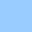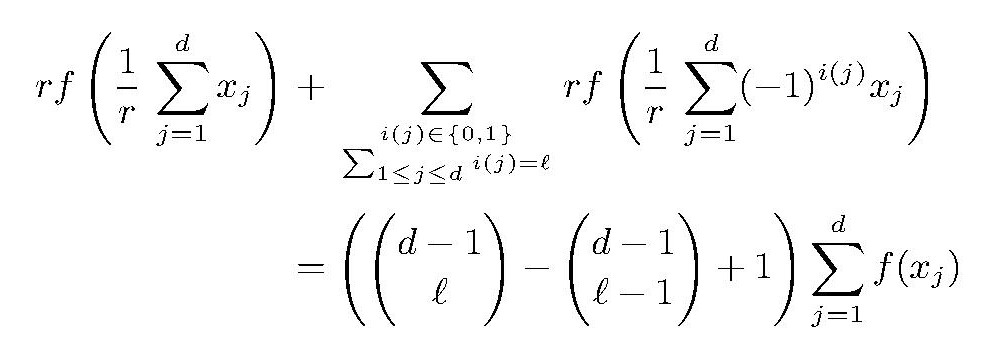On a functional equation by Baak, Boo and Rassias

## On a functional equation by Baak, Boo and Rassias

Jointly written with ANNA BAHYRIYCZ and JENS SCHWAIGER.

Aequationes mathematicae 92, 267–288 (2018). DOI 10.1007/s00010-017-0534-3.

Abstract. In Baak, C., Boo, D.-H., Rassias, Th.M.: Generalized additive mapping in Banach modules and isomorphisms between C*-algebras. J. Math. Anal. Appl. 314(1), 150-161 (2006) the authors considered the functional equationwhere d,ℓ∈ N, 1<ℓ<d/2 and r∈ Q \ {0}.

The authors determined all odd solutions f: X→ Y for vector spaces X,Y over Q. In Oubbi, L.: On Ulam stability of a functional equation in Banach modules. Canad. Math. Bull. 60, 173-183 (2017) the author considered the same equation but now for arbitrary real r not =0 and real vector spaces X,Y. Generalizing similar results from Baak et al. he additionally investigates certain stability questions for the equation above, but as for that equation itself for odd approximate solutions only.

The present paper deals with the general solution of the equation and the corresponding stability inequality. In particular it is shown that under certain circumstances non odd solutions of the equation exist.

harald.fripertinger "at" uni-graz.at, October 10, 2019